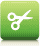# VBScript 变量## VBScript 变量

VBScript 变量名称的规则：

• 必须以字母开头
• 不能包含点号（.）
• 不能超过 255 个字符

## 声明（创建）VBScript 变量

Dim x
Dim carname

carname="Volvo"

Option Explicit
Dim carname
carname=some value

carname="Volvo"
x=10

Dim names(2)

names(0)="Tove"
names(1)="Jani"
names(2)="Stale"

mother=names(0)

Dim table(4,6)

## 实例（仅适用于 IE）

<html>
<body>

<script type="text/vbscript">
Dim x(2,2)
x(0,0)="Volvo"
x(0,1)="BMW"
x(0,2)="Ford"
x(1,0)="Apple"
x(1,1)="Orange"
x(1,2)="Banana"
x(2,0)="Coke"
x(2,1)="Pepsi"
x(2,2)="Sprite"
for i=0 to 2
document.write("<p>")
for j=0 to 2
document.write(x(i,j) & "<br />")
next
document.write("</p>")
next
</script>

</body>
</html>